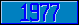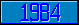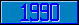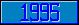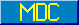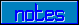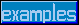## \$%MTXSCA

### M[UMPS] by Example

```
2-Jul–96, 6:54:35

Routine Save for Matrix Mathematics Library function \$%MTXSCA^MATH

;

; Unless otherwise noted, the code below

; was approved in document X11/96–26

;

; If corrections have been applied,

; first the original line appears,

; with three semicolons at the beginning of the line.

;

; Then the source of the correction is acknowledged,

; then the corrected line appears, followed by a

; line containing three semicolons.

;

MTXSCA(A,R,ROWS,COLS,S) ;

; Multiply A[ROWS,COLS] with the scalar S,

; result goes to R[ROWS,COLS]

IF \$DATA(A)<10 QUIT 0

IF \$GET(ROWS)<1 QUIT 0

IF \$GET(COLS)<1 QUIT 0

IF '(\$DATA(S)#2) QUIT 0

;

NEW ROW,COL

FOR ROW=1:1:ROWS FOR COL=1:1:COLS DO

. KVALUE R(ROW,COL)

. SET:\$DATA(A(ROW,COL))#2 R(ROW,COL)=A(ROW,COL)*S

. QUIT

QUIT 1

;===

;

;

;

```